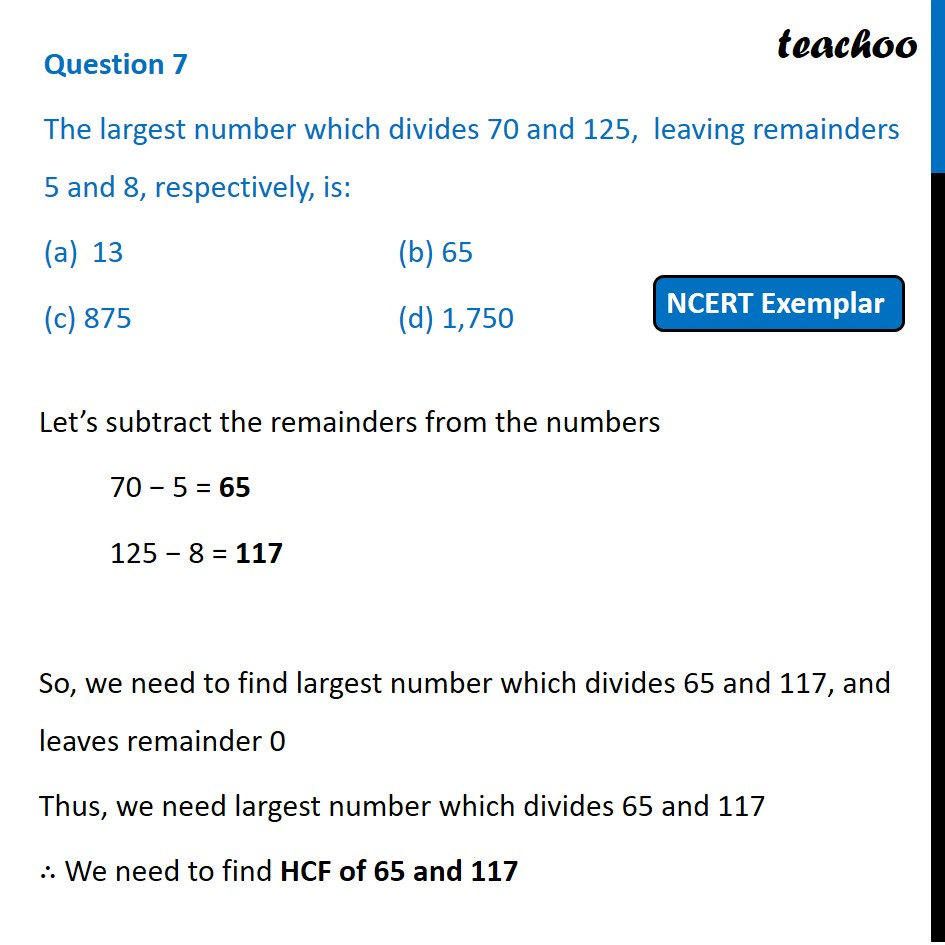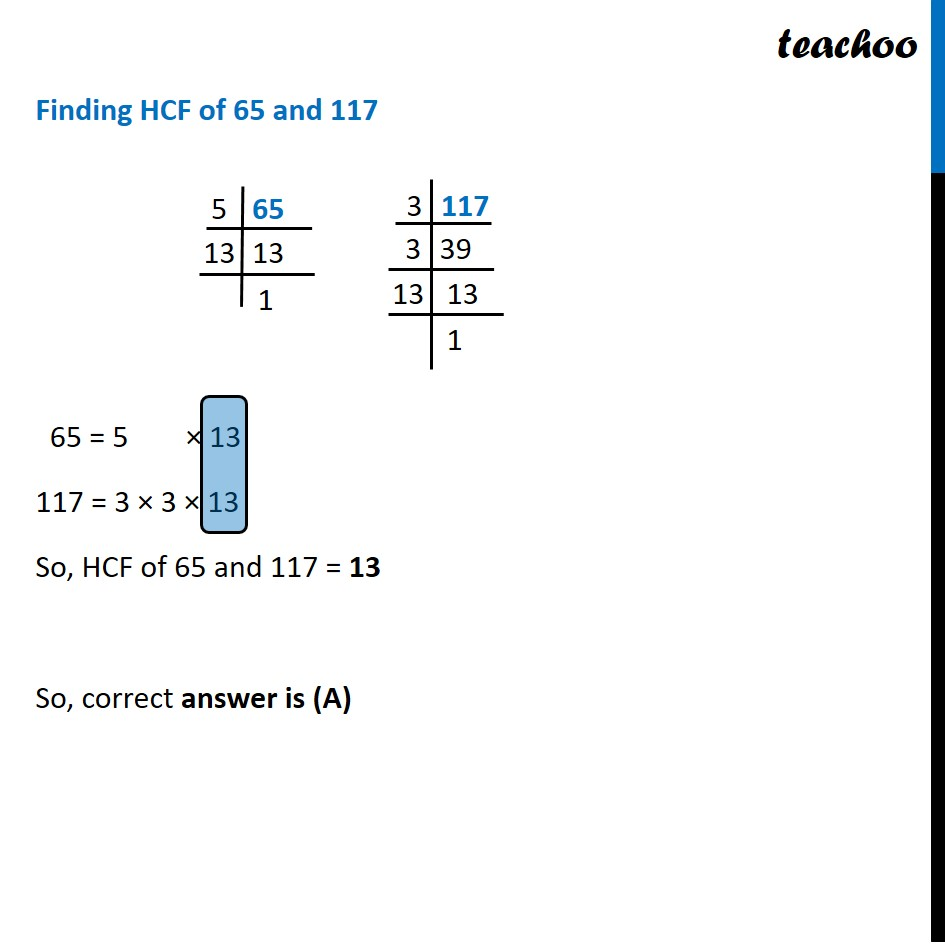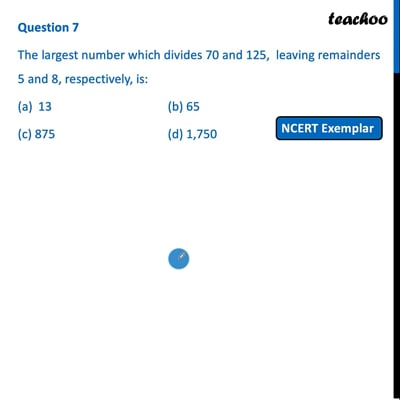MCQs from NCERT Exemplar

Chapter 1 Class 10 Real Numbers
Serial order wise

## (c) 875  (d) 1,750This video is only available for Teachoo black users

Introducing your new favourite teacher - Teachoo Black, at only ₹83 per month

### Transcript

Question 7 The largest number which divides 70 and 125, leaving remainders 5 and 8, respectively, is: 13 (b) 65 (c) 875 (d) 1,750 Let’s subtract the remainders from the numbers 70 − 5 = 65 125 − 8 = 117 So, we need to find largest number which divides 65 and 117, and leaves remainder 0 Thus, we need largest number which divides 65 and 117 ∴ We need to find HCF of 65 and 117 Finding HCF of 65 and 117 65 = 5 × 13 117 = 3 × 3 × 13 So, HCF of 65 and 117 = 13 So, correct answer is (A)